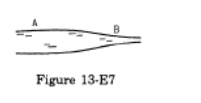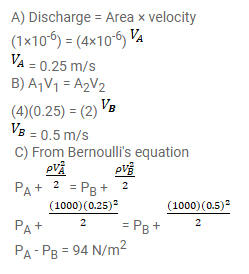# Water flows through a horizontal tube of variable cross-section (figure 13-E7).

Question:

Water flows through a horizontal tube of variable cross-section (figure 13-E7). The area of cross-section at A and B are 4 $\mathrm{mm}^{2}$ and $2 \mathrm{~mm}^{2}$ respectively. If $1 \mathrm{cc}$ of water enters per second through $\mathrm{A}$, find

(a) the speed of water at A,

(b) the speed of water at $B$ and

(c) the pressure difference $P_{A}-P_{B}$.Solution: